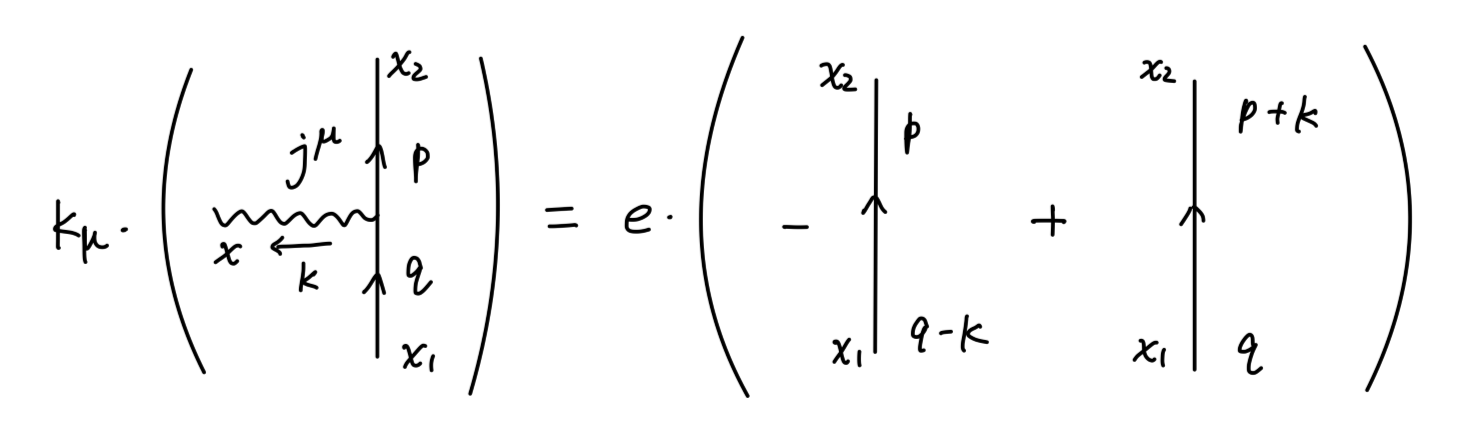## 路径积分表述与对称性

### 运动方程

$\mathcal{L} = \frac{1}{2}(\partial_{\mu}\phi)^2 - \frac{1}{2}m^2\phi^2$

$\langle \Omega| T\phi(x_1)\phi(x_2)\phi(x_3)|\Omega\rangle = Z^{-1}\int \mathcal{D}\phi e^{i\int d^4x \mathcal{L}[\phi]}\phi(x_1)\phi(x_2)\phi(x_3)$

$\phi(x) \rightarrow \phi'(x) = \phi(x) + \epsilon(x)$

$\mathcal{D}\phi' = \mathcal{D}\phi$

\begin{aligned} &\int \mathcal{D}\phi e^{i\int d^4x \mathcal{L}[\phi]}\phi(x_1)\phi(x_2)\phi(x_3)\\ =& \int \mathcal{D}\phi e^{i\int d^4x \mathcal{L}[\phi']}\phi'(x_1)\phi'(x_2)\phi'(x_3)\\ =&\int \mathcal{D}\phi e^{i\int d^4x \mathcal{L}[\phi]}\{\phi(x_1)\phi(x_2)\phi(x_3) + (i\int d^4x \epsilon(x)(-\partial^2-m^2)\phi(x)\phi(x_1)\phi(x_2)\phi(x_3))\\ &\quad + \epsilon(x_1)\phi(x_2)\phi(x_3) + \phi(x_1)\epsilon(x_2)\phi(x_3) + \phi(x_1)\phi(x_2)\epsilon(x_3)\} \end{aligned}

$\epsilon(x_2) = \int d^4x \epsilon(x)\delta(x-x_1)$

\begin{aligned} 0 &= \int \mathcal{D}\phi e^{i\int d^4x \mathcal{L}}[(\partial^2+m^2)\phi(x)\phi(x_1)\phi(x_2)\phi(x_3)\\ &\quad + i\delta(x-x_1)\phi(x_2)\phi(x_3) + i\phi(x_1)\delta(x-x_2)\phi(x_3) + i\phi(x_1)\phi(x_2)\delta(x-x_3)] \end{aligned}

$0 = \int \mathcal{D}\phi e^{i\int d^4x \mathcal{L}}[(\partial^2+m^2)\phi(x)\phi(x_1) + i\delta(x-x_1)]$

$(\partial^2 + m^2)\langle \Omega|T\phi(x)\phi(x_1)|\Omega\rangle = -i\delta(x-x_1)$

$(\partial^2 + m^2)\langle \Omega|T\phi(x)\phi(x_1)\cdots \phi(x_n)|\Omega\rangle = \sum_{i=1}^n\langle\Omega|T\phi(x_1)\cdots(-i\delta(x-x_i))\cdots \phi(x_n)|\Omega\rangle$

\begin{aligned} 0 &= \int \mathcal{D}\varphi e^{i\int d^4x\mathcal{L}}\{(i \int d^4x \epsilon(x)\frac{\delta}{\delta \varphi(x)}\int d^4x'\mathcal{L}) \cdot \varphi(x_1)\cdots \varphi(x_n)\\ &\quad + \sum_{i=1}^{n} \varphi(x_1)\cdots \delta(x - x_i)\cdots \varphi(x_n)\} \end{aligned}\tag{1}

$\frac{\delta}{\delta \varphi(x)}\int d^4x'\mathcal{L} = \frac{\partial \mathcal{L}}{\partial \varphi} - \partial_{\mu}\frac{\partial \mathcal{L}}{\partial (\partial_{\mu}\varphi)}$

$\langle (\frac{\delta}{\delta \varphi(x)}\int d^4x'\mathcal{L}) \varphi(x_1) \cdots \varphi(x_n)\rangle = \sum_{i=1}^n \langle \varphi(x_1)\cdots (i\delta(x - x_i))\cdots \varphi(x_n)\rangle$

$\langle \frac{\delta F[\varphi]}{\delta \varphi}\rangle = -i\langle F[\varphi]\frac{\delta S[\varphi]}{\delta\varphi}\rangle\tag{2}$

### 守恒量与对称性

$\mathcal{L} = |\partial_{\mu}\phi|^2 - m|\phi|^2 \tag{3}$

$\phi(x) \rightarrow \phi(x)' = \phi(x) + i\alpha(x)\phi(x) \tag{4}$

\begin{aligned} \int \mathcal{D}\phi e^{i\int d^4x \mathcal{L}[\phi]}\phi(x_1)\phi^*(x_2) &= \int \mathcal{D}\phi e^{i\int d^4x \mathcal{L}[\phi']}\phi'(x_1)\phi'^*(x_2)|_{\phi' = (1+i\alpha) \phi}\\ &= \int \mathcal{D}\phi e^{i\int d^4x \mathcal{L}[\phi]}\{i\int d^4x[(\partial_{\mu}\alpha)\cdot i(\phi\partial^{\mu}\phi^*-\phi^*\partial^{\mu}\phi)\phi(x_1)\phi^*(x_2)\\ &\quad +[i\alpha(x_1)\phi(x_1)]\phi^*(x_2) + \phi(x_1) [-i\alpha(x_2)\phi^*(x_2)]\} \end{aligned}

$j^{\mu} = i(\phi\partial^{\mu}\phi^*-\phi^*\partial^{\mu}\phi)$

$\langle \partial_{\mu}j^{\mu}\phi(x_1)\phi^*(x_2)\rangle = (-i)\langle(i\phi(x_1)\delta(x-x_1)\phi^*(x_2) + \phi(x_1)(-i\phi^*(x_2)\delta(x-x_2))\rangle$

$\varphi_a(x) \rightarrow \varphi_a(x) + i\epsilon \Delta\varphi_a(x)$

$\mathcal{L}[\varphi] \rightarrow \mathcal{L}[\varphi] + \epsilon \partial_{\mu}\mathcal{J}^{\mu}$

$\mathcal{L}[\varphi] \rightarrow \mathcal{L}[\varphi] + (\partial_{\mu}\epsilon)\Delta\varphi_a \frac{\partial \mathcal{L}}{\partial (\partial_{\mu}\varphi_a)} + \epsilon \partial_{\mu}\mathcal{J}^{\mu}$

\begin{aligned} \frac{\delta}{\delta \epsilon(x)}\int d^4x \mathcal{L}[\varphi + \epsilon \Delta \varphi] &= \partial_{\mu}\mathcal{J}^{\mu} - \Delta \varphi_a \partial_{\mu} \frac{\partial \mathcal{L}}{\partial (\partial_{\mu}\varphi_a)}\\ &= -\partial_{\mu}j^{\mu}\\ \end{aligned}

$j^{\mu} = \frac{\partial \mathcal{L}}{\partial (\partial_{\mu}\varphi_a)}\Delta \varphi_a - \mathcal{J}^{\mu}$

$\langle \partial_{\mu}j^{\mu}\varphi_a(x_1)\varphi_b(x_2)\rangle = (-i)\langle (\Delta \varphi_a(x_1)\delta(x-x_1)\varphi_b(x_2)) + (\varphi_a(x_1)(\varphi_b(x_2)\delta(x-x_2))\rangle$

### Ward 等式

$\psi(x) \rightarrow (1+ie\alpha(x))\psi(x)$

$\mathcal{L} \rightarrow \mathcal{L} - e\partial_{\mu}\alpha\bar{\psi}\gamma^{\mu}\psi$

\begin{aligned} 0 &= \int\mathcal{D}\bar{\psi}\mathcal{D}\psi \mathcal{D}A e^{i\int d^4x \mathcal{L}}\{-i\int d^4x \partial_{\mu}\alpha(x) [j^{\mu}(x)\psi(x_1)\bar{\psi}(x_2)]\\ &\quad + (ie\alpha(x_1)\psi(x_1))\bar{\psi}(x_2) + \psi(x_1)(-ie\alpha(x_2)\bar{\psi}(x_2)) \} \end{aligned}

$j^{\mu} =e \bar{\psi}\gamma^{\mu}\psi$

\begin{aligned} i\partial_{\mu}\langle 0|Tj^{\mu}(x)\psi(x_1)\bar{\psi}(x_2)|0\rangle &= -ie\delta(x-x_1)\langle 0|T\psi(x_1)\bar{\psi}(x_2)|0\rangle\\ &\quad + ie\delta(x-x_2)\langle 0|T\psi(x_1)\bar{\psi}(x_2)|0\rangle\\ \end{aligned}\tag{5}

$\int d^4x e^{-ik\cdot x}\int d^4x_1 e^{+iq\cdot x_1} \int d^4x_2 e^{-ip\cdot x_2}$$-ik_{\mu}\mathcal{M}^{\mu}(k;p,q) = -ie\mathcal{M}_0(p;q-k) + ie\mathcal{M}_0(p+k;q)$